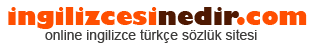ingilizce türkçe sözlük sitesine hoşgeldiniz.| Ana Sayfaİngilizce Türkçe Sözlükte Ara: Türkçe->İngilizce İngilizce->Türkçe * Aramak istediğiniz kelimeyi yazıp dil seçimini seçtikten sonra kelimenin anlamını öğrenebilirsiniz.

Kelime Anlamı

## Türkçeden İngilizceye Çeviri Sonucu

### megahertz Anlamı

• megacycle , megahertz.
• A measure of frequency in millions of cycles per second Can be used to compare relative speed of CPUs which carry out the same number of operations per cycle but meaningless in comparing different types of CPUs. one million periods per second.
• One million cycles per second; a measure of a computer's speed - how fast it can internally process commands.
• A million cycles per second, abbreviated MHz This is often used misleadingly to indicate processor speed, because while one might expect that a higher number would indicate a faster processor, that logic only holds true within a given type of processors as different types of processors are capable of doing different amounts of work within a cycle For a current example, either a 200 MHz PowerPC or a 270 MHz SPARC will outperform a 300 MHz Pentium.
• Unit of frequency equal to 1,000,000 Hz.
• One million cycles per second A unit of measure for frequency.
• The unit of measurement of the speed of the microprocessor Literally one million cycles per second.
• A unit of frequency denoting one million hertz, or one million cycles per second.
• One million cycles per second A measurement of microprocessor chip oscillation speed. Measurement of wave or clock frequency, one million cycles per second.
• A unit of frequency equal to 1,000,000 cycles per second. a frequency of one-million Hertz, or 106 cycles per second. - a unit of frequency equivalent to one million 'cycles-per-second' One Megahertz equals 1,000,000 cps. a frequency measure corresponding to a signal that oscillates at one million cycles per second Megahertz is often used when referring to the clock speed of a computer.
• Millions of cycles per second The transmitter at a radio station with a frequency of 91 5 MHz, therefore, is oscillating at 91,500,000 cycles per second.
• One million hertz.
• One million cycles per second Used to describe a radio frequency.
• A measurement of clock cycles in millions of cycles per second. One million cycles per second.
• A measurement of frequency equal to one million cycles per second One cycle per second is one hertz.
• One million Hertz or 1,000,000 cycles per second.
• One million cycles per second. 1 million cycles per second.
• A million hertz; a million cycles per second.
• A frequency of one million cycles per second.
• megahertz. megaherts megacycle.
• one million periods per second.
Rastgele Türkçe Kelimeler